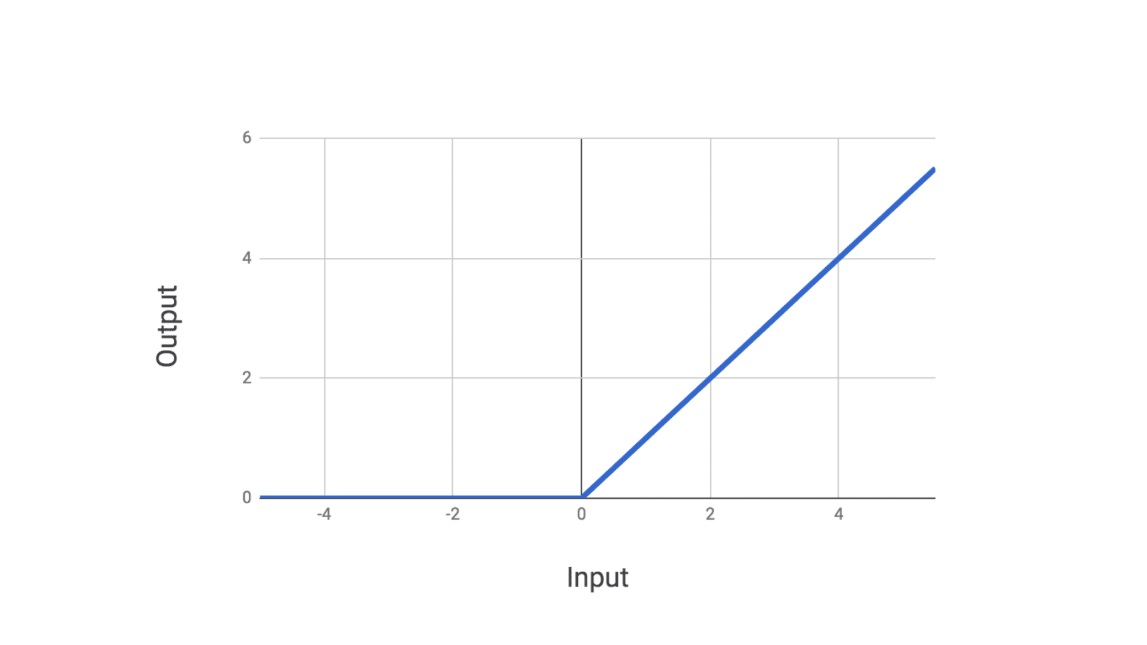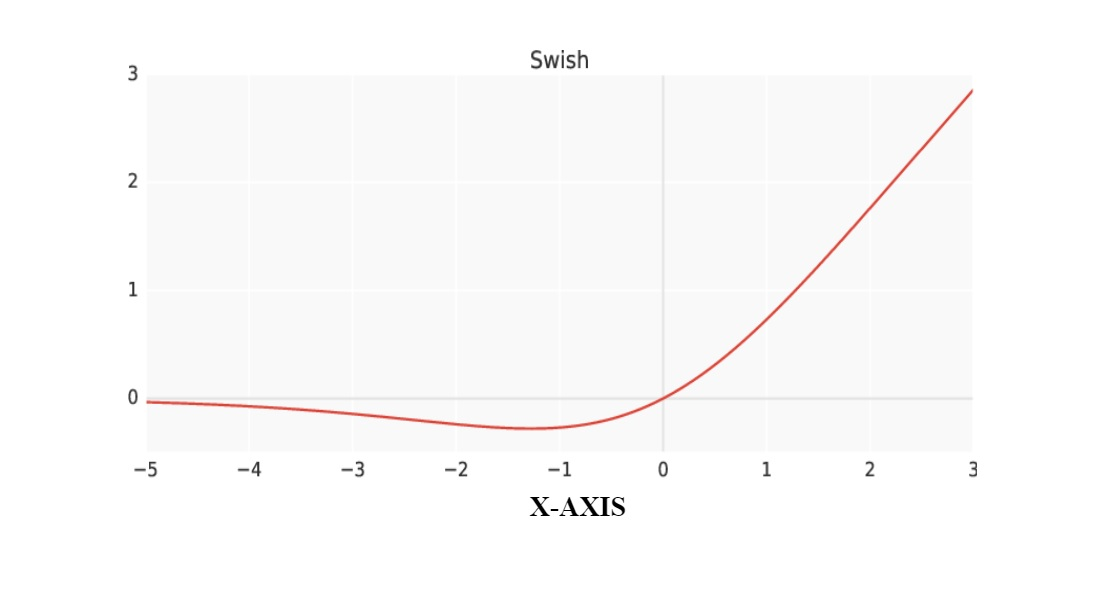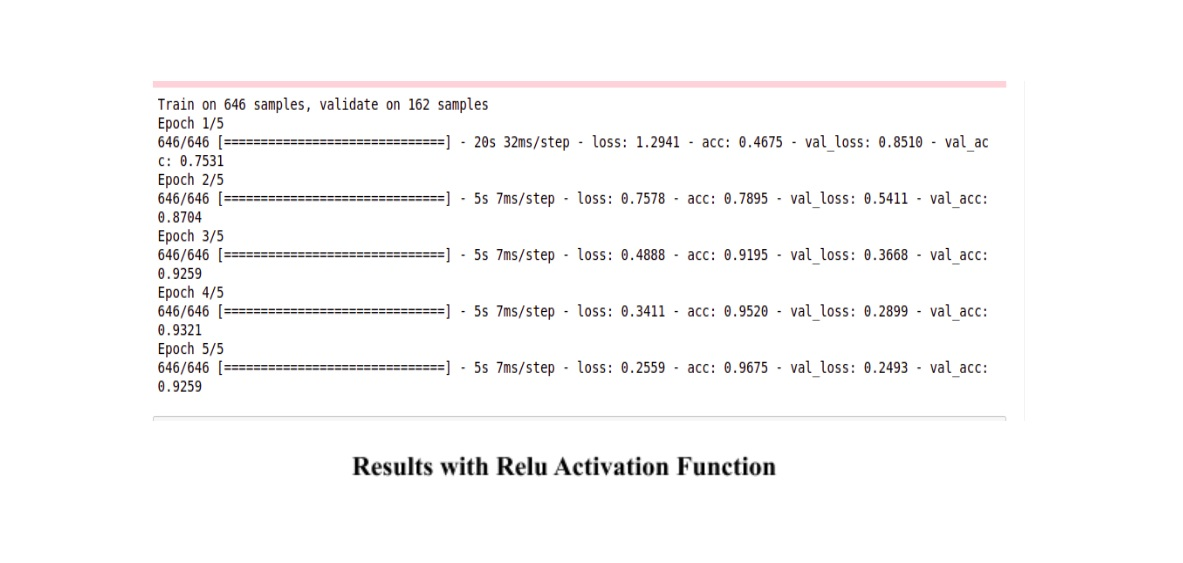# Swiss Activation Function

## Swish Activation Function

• 360 DT
• 2 June, 2020

### A new method to Increase the Accuracy of the Machine Learning Models using Swish Activation Function

If you are new to machine learning you might have heard about Activation functions but not sure how they work in the input layers. Let’s do a fast recap about what is an Activation Function?

#### What is an Activation Function?

Activation Function: The activation function of a node in the artificial neural network defines the output of the node given the input or a set of inputs. It is a non-linear Mathematical Transformation that we do in the input before sending it to the Output Layers. It is a sum of all the inputs and a bias is added to the function and then the function decides whether the neuron should be fired in the neural network or not.

Y=weight*input(x) + Bias

Where, Y is a neuron in the neural network

have a range between (-infinity,infinity)

In the above equation, the neuron does not know about the range of the value. So we decide whether a neuron should be fired or not with the help of activation function.

A neural network works in the same way our brain works. What type of complex mathematics is behind the working of activation functions. Let’s take an example for Relu activation Function

Let us discuss why the Swish Activation Function was created?
RELU

ReLu = 0, for x<0

ReLu = max(0,x),for x>=0

The above equation represents the relu and it shows that Relu is positive when x>0 and Relu is 0 for any negative value in the function
Interactions: Imagine a single node in a neural network model. For simplicity, assume it has two inputs, called X and Y. The weights from X and Y into our node are 2 and 3 respectively. So the node output is F(2X+3Y). We will use the Relu Function for our function F.

If(2X+3Y) is positive, then the output of our node is also Positive.
If(2X+3Y) is negative, then the output of our node is 0.The above graph shows the graphical representation of Relu Activation.

What is the problem with Relu?

The problem for Relu arises when the value of x is negative because for negative value x is 0. When we use a Machine Learning model with multiple negative input values it brings those values to 0 which causes a loss of information in our model. In Machine Learning after every epoch, we learn from our errors at the end of our forward path, during the backward propagation of the neural network we update the weight and bias of our network to make better predictions every time an epoch is run. What happens during the backward propagation between two neurons out of which one is a negative number close to one and the other one is a large number. There will be no method to know one was closer to 0 than the other neuron in the forward propagation because we have removed the information. Once the negative value reaches 0 it is rare for the weight to recover in the upcoming forward pass and this leads to the Dying RELU Problem.

This problem stated above has been resolved in October 2017, Prajit Ramachandran, Barret Zoph, and Quoc V. Le from Google Brain proposed the Swish enactment work. This new enactment work expands the exactness of the CNN structures by 1 percent. This new enactment work expanded the precision of various exchange learning calculations like InceptionNet, Resnet, Imagenet, and so on and so forth.

#### SWISH Activation Function

This is an alternative to the older Activation Function Relu and outperforms Relu by a 1 percent increase in the accuracy of the top Transfer learning algorithms like InceptionNet, VGG16, DenseNet, Mobile net, and many others. It has performed significantly better in the deeper Neural Networks. It is also known as a self gated function.

(x)=x*sigmoid(x)

(x)=x*11+e-x

The above equation represents the Mathematical Equation of Swish Activation Function.The above graphical representation shows that Swish is smooth and non-monotonic(not increasing or decreasing in the entire domain). Swish is better because it is non-monotonic, unbounded above, and bounded below are all advantageous.

Swish however diverges from ReLU because it builds outputs for small negative inputs due to its property of non-monotonicity. It upgrades the flow of gradient descent, which is important considering that many activations fall into this scope. Moreover, the uniform flatness of the curve plays an important role in optimization.

It is the same as the activation function Relu except that the domain around 0 differs from Relu. Swish does not change its value like the Relu does instead , it bends for negative values of x and for x>0, it increases upwards. Swish Function outperformed all the other activation functions like (Relu, Tanh, Sigmoid) for deeper neural networks.

How to use the Swish Activation Function in your Machine Learning Model?

There is no direct implementation of Swish in Keras Library. So First we have to make a basic python function for Swish.

CODE:

1.from keras.import sigmoid       //Sigmoid is imported from keras library

2.def swishfunction(x,beta=1)          //Function is created
return (x*sigmoid(beta*x))

Next, we register the custom objects using Keras and tell to update it and then pass it to a dictionary with a key with which we will call our Swish Function

3.from keras.utils.generic_utils import get_custom_objects from keras.layers import Activation
4.get_custom_objects().update({‘swishfunction’:Activation(swish)})

Finally, We can use Swish Function in the input Layers as shown below
6.model.add(Dense(256, activation = “swishfunction”))        //Using Swish function
7.model.add(Dense(128, activation = “swishfunction”))        //Using Swish function in Dense Layer

In the Last Layer

#### Swish is a better activation function than Relu because:

1. It is a smooth curve because of which it will learn the properties of the neural network better than Relu and outperforms as it is a Non-Monotonic function.
2. Since Relu does not perform for negative values as Relu (f(x)=0 for x<0) and sometimes negative values are also important characteristics of the neural network in predicting the patterns . Relu leads to loss of information whereas Swish does the opposite. It helps the model in learning the negative inputs and gives a better result.

So much theory! Right Let’s get into some code
I have applied CNN with ResNet50 on a dataset consisting of images of humans, cats, dogs, and horses.

You can get the dataset from the link given below:
https://www.kaggle.com/eabdul/dogs-cats-horses-humans-dataset/discussion

I trained my Model twice using the same algorithms and keeping all the values same of all the hyperparameters in both the cases. But in the first case I have used Relu as an activation function while in the second case I have used the new activation function Swish .As expected the accuracy was higher in the case of Swish. The Accuracy obtained was 97.37% in the case of Swish while the accuracy was 96.75% for Relu.

#### You can see the results mentioned below in the images:You can check out our Github profile for the above Source code at the link given below:
https://github.com/360DT/Swish-Activation-Function

#### Written By:

Inderpreet Singh Walia